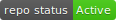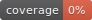# L2boost PackageThe L2boost package is an efficient implementation of Freidman’s boosting algorithm for linear regression using an l2-loss function and coordinate direction (design matrix columns) basis functions. The package was developed in support of Ehrlinger and Ishwaran (2012)

The l2boost package implements a generic boosting method [Friedman (2001)] for linear regression settings using an l2-loss function. The basis functions are simply the column vectors of the design matrix. l2boost scales the design matrix such that the boosting coefficients correspond to the gradient direction for each covariate. Friedman’s gradient descent boosting algorithm proceeds at each step along the covariate direction closest (in L2 distance) to the maximal gradient descent direction.

The l2boost function uses an arbitrary L1-regularization parameter (nu), and includes the elementarydata augmentation of Ehrlinger and Ishwaran (2012), to add an L2-penalization (lambda) similar to the elastic net [Zou and Hastie (2005)]. The L2-regularization reverses repressibility, a condition where one variable acts as a boosting surrogate for other, possibly informative, variables. Along with the decorrelation effect, this elasticBoost regularization circumvents L2Boost deficiencies in correlated settings.

We include a series of S3 functions for working with l2boost objects: * print (print.l2boost) prints a summary of the fit, * coef (coef.l2boost) returns the model regression coefficients. * fitted (fitted.l2boost) returns the fitted response values from the training set, * residuals (residuals.l2boost) returns the training set residuals, * plot (plot.l2boost) for graphing, * predict (predict.l2boost) for prediction on possibly new observations,

A cross-validation method (cv.l2boost) is also included for L2boost and elasticBoost cross-validating regularization parameter optimizations.

### Example Datasets

We have repackaged thediabetes data set from Efron et. al. (2004) for demonstration purposes. We also include simulation functions for reproducing the elastic net simulation (elasticNetSim) of Zou and Hastie (2005) and the example multivariate normal simulations (mvnorm.l2boost) of Ehrlinger and Ishwaran (2012).

Friedman J. (2001) Greedy function approximation: A gradient boosting machine. (Ann. Statist.), 29:1189-1232

Ehrlinger J., and Ishwaran H. (2012). “Characterizing l2boosting” (Ann. Statist.), 40 (2), 1074-1101

Zou H. and Hastie T (2005) “Regularization and variable selection via the elastic net” (J. R. Statist. Soc. B), 67, Part 2, pp. 301-320

Efron B., Hastie T., Johnstone I., and Tibshirani R. (2004). “Least Angle Regression” (Ann. Statist.) 32:407-499